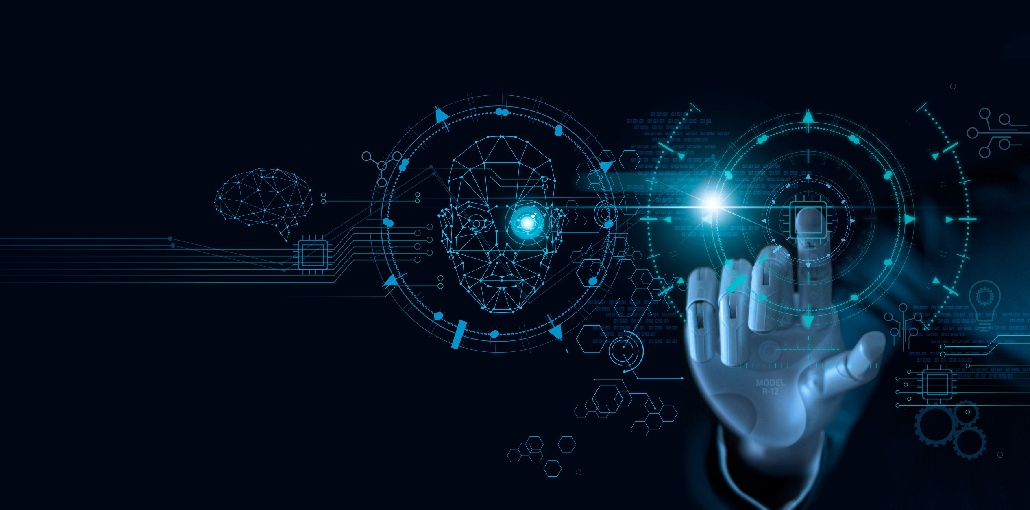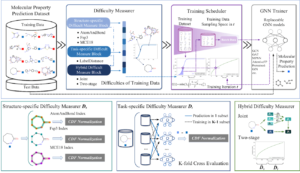# How to Use Curriculum Learning to Build a Robust ML Model# How to Use Curriculum Learning to Build a Robust ML Model

Every machine learning program has a learning process that is at least partially influenced by the learning behavior and learning style of humans.

An image classification model, for example, tells us the class of an image like a person using the knowledge that humans have provided in the form of data.

Another type of machine learning is curriculum learning. It trains the model so that humans can learn from their education system.

## What is curriculum learning?

One type of machine learning process that can be called curriculum learning is one that is inspired by the human learning strategy. All other machine learning techniques are also inspired by human learning strategies, but this learning is a way to make machines learn gradually. We all know that humans learn best by using simple examples, and then we can understand more complex examples. This type of learning uses simple to complex examples to help a machine learn about any task.

This learning can be described as the process of training machine-learning models by using simpler tasks or parts of the task and then gradually increasing the complexity. This type of learning can be illustrated by making a machine learn grammar architectures. We can push a limited amount of grammar knowledge into a recurrent neural system at the beginning. However, as the network learns more, we can increase our resources for learning. This learning strategy is crucial for machines that are developing psychology.

Also read: Getting Started with Classification in Machine Learning

## Effectiveness of curriculum learning

Large datasets are a common feature of machine learning processes. While these datasets are sufficient to make a machine learn, the problem lies with the models. Models that are poorly parameterized can lead to higher levels of error and loss. When we expect higher accuracy from our models, it can be difficult to model whole data at once. There are also issues such as slow modeling, failure of convergence, and others. These are just a few of the problems we have with general modeling.

These points can be used to say that learning is a process in which the complexity of the examples is increasing and the data we provide is slowly increasing. If we take a look at the data that we provide to the model, it may consist of very few examples or samples. This allows us to see changes in robustness and convergence.

The model can be easily learned by using simple examples. The likelihood of improving the accuracy of the model is higher when the data are being pushed out gradually. This type of learning is more effective than traditional methods. Let’s take an example of real-life animal training. The animal can be confused if it is not given the opportunity to learn all the information at once. However, if the knowledge is applied with increasing difficulty the animal can be trained for any task.

## A simple example of curriculum learning

This section will explain how to proceed with curriculum learning. Let’s suppose that we have created a convolutional neural system. This network is composed of convolutional layers, a fully connected layer with neurons, and an output layer. This type of network is difficult to train with high-dimensional data. It can also become bound to give accuracy up to 50 to 60%.

Let’s look at the CIFAR-10 data. We know there are 10 classes in the CIFAR-10 data. Some classes are more difficult to learn. This scenario allows us to train our model with classes that are simple to learn. Six classes out of a total of 10 are easy to learn. We can then start training with 6 classes, and then introduce new classes at each epoch. After 5 epochs, the model will be able to learn in each class. We can then train a machine-learning model in the curriculum way.

Below is an illustration of how a model uses the curriculum learning method for molecular graph learning.Image Source: GitHub

The image above is easy to understand. Nevertheless, we can still see the idea of creating blocks in our curriculum learning process by simply looking at it.

## Use cases of curriculum learning

There are many uses for curriculum learning. Here are some examples:

There are five types of curriculum learning programs that can be used in reinforcement learning.

• Teacher-student curriculum learning
• Self-play curriculum learning
• Goal generation curriculum learning
• Skills extraction curriculum learning
• Distillation curriculum learning

We can see how important reinforcement learning is by just looking at this type. All types of curriculum learning follow the same basic principle: increase the complexity of the learning samples and the environment for an agent.

Also read: Top 10 Machine Learning Platforms in 2022

• This type of learning is used in chemistry to learn about the structures of molecules and compounds.
• There are many neural networks that can perform classification. They can also include curriculum learning to increase their accuracy.
• This type of program can be used in projects where data is not balanced. This type of learning can be called continuous learning.
• This type of learning algorithm can be implemented in many ways. that can be found in the industry (answering questions ) and other industries that assist us in improving our content’s grammar.
• We can use curriculum learning in the field of natural language processing to improve the performance and quality of neural machine translation.

## Last words

We have talked about curriculum learning, which can be used to model better general models. This is done by pushing data into training and increasing the complexity of the data samples gradually. We also discussed the effectiveness, examples, and uses of curriculum modeling.